# Distance between randomly sampled points in sphere using MATLAB

March 18, 2022

lang: en_US

Random sampling within a sphere is a process used in several fields such as mathematics and spatial statistics, ecology, astronomy, economics, and particle physics, to name a few.
In statistics, point processes in stochastic geometry use a set of random sample points in a given mathematical space such as spheres, cubes and even generalized to n-dimensional spaces. In this blog, the distribution of pairwise Euclidean distances between random points in a unit sphere is described using random sampling by the inverse CDF transform method.

## The preferred approach to random sampling

There are different ways to generate random samples within a mathematical space described in the literature. In this blog, the approach discussed is based on the inverse CDF method. This algorithm is preferred over the usual approach of finding random points. Simply scaling the normalized position vector by a uniform random number causes the points to be concentrated within the sphere's center. In this algorithm, the scaling is done with the cube root of the uniform random number instead of just the number, which causes the points to be more spread out in the sphere. The connection of this algorithm with the probabilistic interpretation is also provided to serve as a basis for this algorithm.Vector_normrandom-points-sphere

## Algorithm

Firstly, the process of random sampling involves generating the set of random points within a unit sphere. The coordinates of a point are chosen from a normal distribution with mean 0 and variance 1. This vector is then normalized to produce a unit vector that specifies the direction along which the random point is obtained in the sphere's interior. After this, mapping the point on the sphere to produce a point within the sphere is done by scaling the normalized vector using a number chosen from a uniform distribution for the radius. These are effectively the position vectors of the random points within the unit sphere, also referred to as radii. Then the pairwise Euclidean distances between these randomly sampled points are obtained, and the distribution of these distances is observed.

## Theoretical foundation

The theory behind this approach is that the infinitesimal volume of a sphere depends on a randomly chosen radius 'r' coupled with a random point on the surface of the unit sphere. The infinitesimal volume is proportional to the square of the radius 'r'. The sampling density needs to match the desired distribution. For this, the probability density function 'pdf' and cumulative density function 'CDF' of the target distribution are specified. However, it is not enough to have the pdf and CDF if we cannot produce random variables to match this distribution. So, a process known as probability integral transform is done to map/transform a uniform random variable to get a random point coordinate within the sphere.

The following equation is used –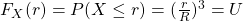where P is the probability of the distance of the point from the center 'X' being less than or equal to 'r'. This is also known as cumulative distribution function 'F(r)'. Hence 'r' can be obtained as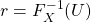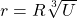where U is the uniform random variable from 0 to 1

The random point thus generated is given by,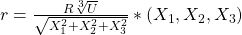where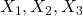are independent and identically distributed (iid) standard normal variables for the direction along which the random point is to be mapped and is independent of U.
Then the pairwise Euclidean distances between sample points are obtained, and the resulting histogram is computed.Probability Integral Transform

### MATLAB Codes for distribution of pairwise distance by random sampling

%%%%%%%%%%%%%%%%%%%%%%%%%%%%%%%%%%%%%
function r = sample_point_sphere ()
% Returns sample points in the unit ball by the transformation of the
% uniform random variable to a random sample point within the sphere.
% This is done by normalizing three normally distributed random variables
% X1, X2, X3 with mean 0 and variance 1 for the direction along which the point is % to be obtained.
% Then this vector is scaled by the cube root of a uniformly chosen random variable

% Author: Anuradha Viswanathan, MATLAB Helper
% Topic: Random distance sampling (generating sample points)
% Website: https://matlabhelper.com
% Date: 03-02-2022

R = randn (3,1);
% Normalize the vector.
R_unit = R/norm(R);
% compute a value to map the point ON the sphere INTO the sphere.
u = rand ();
r = u^(1/3)*R_unit;

return
end

function distance_sphere(n)
% This script takes as input the 'n' number of pairwise sample points. It finds the
% pairwise distances between randomly sampled points
% in a sphere and displays the histogram as well as a unit sphere containing
% red lines representing the pairwise distances

% Author: Anuradha Viswanathan, MATLAB Helper
% Topic: Random distance sampling
% Website: https://matlabhelper.com
% Date: 03-02-2022

clc

figure (1) %creating animation window
sphere % creating sphere for visualization
alpha('clear') % making sphere clear for visualization
hold on; % make it so that all animations are drawn on same figure
for i = 1 : n
% Finds 2 sample points
p = sample_point_sphere();
q= sample_point_sphere();
vec = [p';q'];
% Draws a line connecting the 2 sample points inside the sphere
plot3(vec(:,1),vec(:,2),vec(:,3),'LineWidth', 2, 'Color', 'r');
hold on
% Finds the Euclidean distance between the points
t(i) = norm(p - q);
end
hold off
figure(2)
histogram(t,'Normalization','probability');
grid ('on')
xlabel('Distance')
ylabel('Probability')
title('Distance between a pair of random points in a unit ball')
hold off
return
end

### Output generated by the code

The below figures show the output generated by the code. The first figure shows the unit sphere with random internal points within it and connected by red lines representing pairwise distances between the points. The second figure is the histogram of pairwise distances between ‘n’ sample points generated within the sphere.distances-in-spherehistogram-pairwise-distances

## Conclusion

The algorithm for finding the distribution of pairwise distances between randomly sampled points is presented along with the MATLAB code in the above sections. Some theoretical concepts were applied in this code. The advantages of using this approach were also highlighted.

Ready to take your MATLAB skills to the next level? Look no further! At MATLAB Helper, we've got you covered. From free community support to expert help and training, we've got all the resources you need to become a pro in no time. If you have any questions or queries, don't hesitate to reach out to us. Simply post a comment below or send us an email at [email protected].

And don't forget to connect with us on LinkedIn, Facebook, and Subscribe to our YouTube Channel! We're always sharing helpful tips and updates, so you can stay up-to-date on everything related to MATLAB. Plus, if you spot any bugs or errors on our website, just let us know and we'll make sure to fix it ASAP.

Ready to get started? Book your expert help with Research Assistance plan today and get personalized assistance tailored to your needs. Or, if you're looking for more comprehensive training, join one of our training modules and get hands-on experience with the latest techniques and technologies. The choice is yours – start learning and growing with MATLAB Helper today!

Education is our future. MATLAB is our feature. Happy MATLABing!

MATLAB Developer at MATLAB Helper,
M.S in Telecommunications and Networking,
M.S in Physics

#### MATLAB Helper ®

Use Website Chat or WhatsApp at +91-8104622179